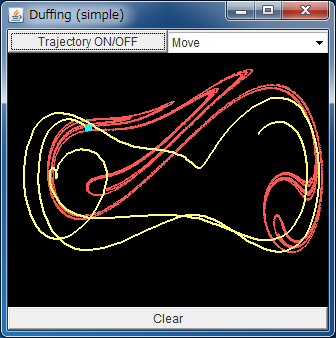# Attractor of Duffing EquationIf the above application does not start, please install OpenJDK from adoptium.net.

Here we treat Duffing equation written as

d2x /dt2 = - δ dx/dt + x - x3 + γ cos(ω t),

where δ=0.20, γ=0.30, ω=1.0. This model describes the dynamics of a periodically driven point mass in a double-well potential. A Japanese engineer Dr. Yoshisuke Ueda had used the slightly modified version of this Duffing equation when he found chaos in his model.

The Duffing equation is very simple, which is obtained by adding a nonlinear term x3 to a linear differential equation; therefore, it is often treated in the textbooks of nonlinear dynamics.

The The yellow trajectory shows the dynamics of (x, dx/dt). The point mass seems to fluctuate randomly between bottoms of two wells, but, actually, it obeys a deterministic rule.

Embedding this solution to the three dimensional space (x, dx/dt, t) and cutting the trajectory with a plane,
you can see the periodical motion of chaos attractor like "Duffing equation" animation.
This simulator shows the same movement of the attractor with a red color.

You can find that the states of the point mass stays on (or, is attracted to) the chaotic attractor. This is an example which shows "order within chaos".

You can give a new set of initial conditions by clicking the field.